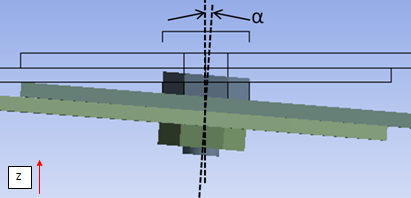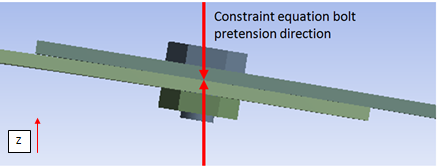you are here:/ News & Insights / Engineering Advantage Blog / Why Shouldn't I Use Constraint Equations in a Structural Analysis with Large Deformations?

# Why Shouldn't I Use Constraint Equations in a Structural Analysis with Large Deformations?July 18, 2014 By: Patrick Cunningham

You may have heard the disclaimer in CAE Associates' ANSYS update seminars, training classes and e-Learning webinars: be careful about using constraint equations in a large deformation analysis. Well, what's that all about? Constraint equations are a very convenient way to connect and/or define relative motion between bodies. They allow us to create cyclic and repetitive symmetry boundaries. They provide a way to control the displacement of a collection of nodes all tied to a single master node. Best of all they do all these useful things while reducing the size of the mathematical problem by removing degrees of freedom from the model. Why then are they a cause for concern in large deformation analyses?

First let's talk about what happens when we include large deformation effects in our finite element analysis models.  Large deformation, a type of geometric nonlinearity, includes the following items:

• Updating certain load vectors (pressure, for instance) to account for the deformation of a structure. These are so-called follower forces.
• Updating the stiffness of elements experiencing significant deformation (large strain effects) or rigid body rotation.
• Considering the deformed shape in the calculation of stress. The change in the length of the moment arm in a beam under a bending load is a typical example of this.
• Including stress-stiffening effects that result from loading like the membrane or in-plane stiffness of a thin structure subjected to an out of plane load.

One thing that is typically not modified during this process is the orientation of the nodal coordinate systems. These remain in their initial orientation as the structure undergoes large rotations, which is the key reason why we need to be careful when using constraint equations in a large deformation analysis. The constraint relation is defined in the original nodal coordinate system, which does  not change as the structure deforms or experiences large rotations.

Consider the bolted connection between two flexible plates shown in Figure 1. It is common practice to define a bolt preload using constraint equations. These equations are written at the beginning of the analysis using the original nodal locations and orientations. If we assume that the Z-direction is along the undeformed axis of the bolt, the preload will be produced via constraint equations relating the Z-deformations of bolt nodes. Whether or not this approach is valid with large deformation will depend on the amount of rotation that the bolt axis experiences as the plates deform.  In a small deformation solution we assume that the angle α between the deformed and undeformed bolt axis is negligible.If the bolt experiences large rotation, the bolt preload direction needs to be updated with respect to the current bolt axis. However, when a constraint equation preload is used, the pretension direction remains in the undeformed Z-axis direction as illustrated in Figure 2 below. For a large rotation angle, α, the error in preload direction could cause significant error in the results.The good news is that if your bolt preload is applied in regions of the model that are not undergoing large rotations, they will still be valid. For this reason, it is important to check your initial results carefully.  If you do see large rotations of the bolts in preloaded regions there are other ways to apply bolt preload correctly. One approach is to use a multi point constraint (MPC) with preload capabilities in place of the actual bolt geometry, if your FEA software supports it. This is a more advanced form of a constraint equation that will update with large deformation.

There are many other approaches to this type of connection.  Does anyone have a method they would like to share?